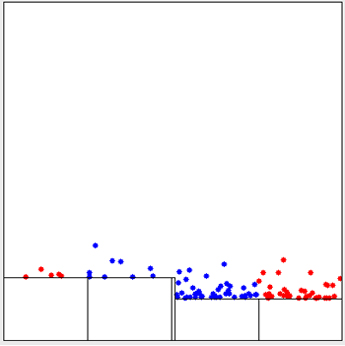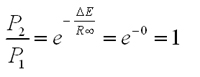# Entropy Activity

-Introduction

-The reaction coordinate

-The energy landscape

-Population distributions

-Motion at constant temperature, and exchange of energy with the heat bath

-Thermally activated processes

-Energy and temperature determine the populations

-Entropy and free energy

-Mathematical derivation of free energy

 < Previous | Next >

## Energy and temperature determine the populations

Once a system reaches thermal equilibrium, the relative populations of two states is related only to the difference in energy between the states, ΔE, and the temperature T, through the relation P2/P1 = e-ΔE/kT. The details of the dynamics do not play a role. This next activity illustrates this concept.

Virtual Activity

- Energy and temperature determine the populations [Opens in a separate window.]

Now consider the following two arrangements of platforms. The energies of the platforms are the same in both arrangements, but the order of the platforms is different.Arrangement 1Arrangement 2

Question: Will the populations of the upper and lower energy platforms be the same for both arrangements?

Consider the following two arrangements of platforms (The difference in energy between the platforms is the same in both arrangements. In the arrangement 2, both platforms have been raised to a high energy.)Arrangement 3Arrangement 4

Question: At thermal equilibrium, with the ratio of populations between the higher and lower platforms (P2/P1) be the same for both arrangements?

At thermal equilibrium, the ratio between the populations of any two individual platforms is given by:Comprehension Question: Is there a temperature for which a higher energy platform can have a larger population than a lower population, i.e. can P2/P1 >1 when E2>E1?

Answer: No. At low temperature, there will be more particles on the lower-energy platform. As we heat the system up, the population of the upper platform increases. At an infinitely high temperature, the population of the higher-energy platform will become equal to that of the lower-energy platform (P2/P1 = 1), but P2/P1 will never be greater than one. This can be seen mathematically as:Where we have used the fact that< Previous | Next >